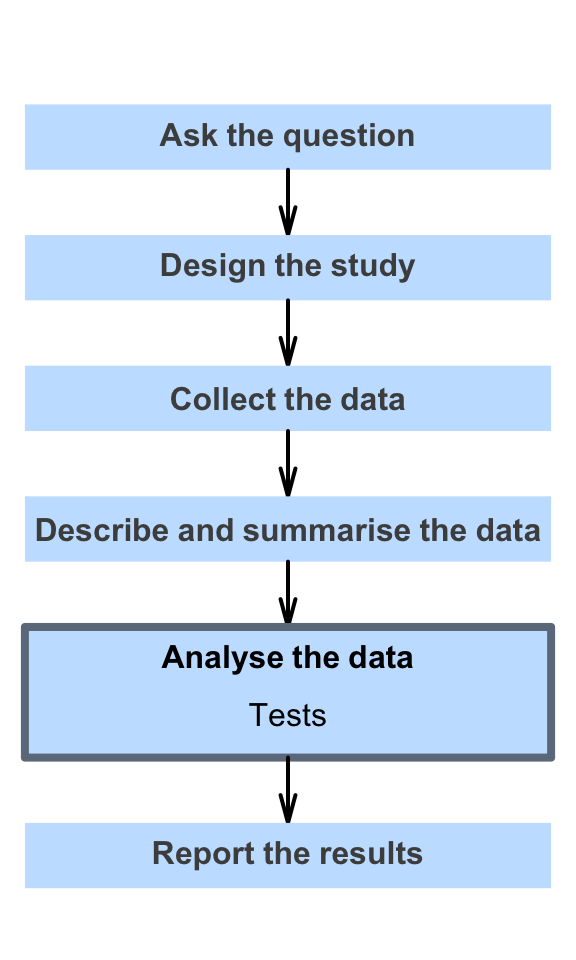# 28 More about hypothesis testing

So far, you have learnt to ask a RQ, identify different ways of obtaining data, design the study, collect the data describe the data, summarise data graphically and numerically, understand the tools of inference, and to form confidence intervals.

In this chapter,
you will learn about hypothesis tests. You will learn to:

• communicate the results of hypothesis tests.
• interpret $$P$$-values.